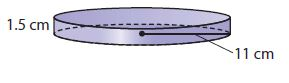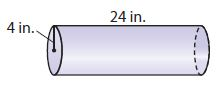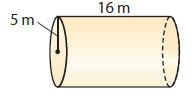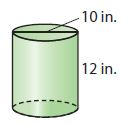Homework Explained - Math Practice 101Dear guest, you are not a registered member. As a guest, you only have read-only access to our books, tests and other practice materials.

As a registered member you can:

Registration is free and doesn't require any type of payment information. Click here to Register.
Go to page:
Chapter 13:Volume; Lesson 1: Volume of Cylinders

13.1 Independent Practice

Find the volume of each figure. Round your answers to the nearest tenth if necessary. Use 3.14 for $$\pi$$.

• Question 6•  $$\text{ cm}^3$$
• Question 7•  $$\text{ in}^3$$
• Question 8•  $$\text{ m}^3$$
• Question 9•  $$\text{ in}^3$$
• Question 10

A cylinder has a radius of 4 centimeters and a height of 40 centimeters.

•  $$\text{ cm}^3$$
• Question 11

A cylinder has a radius of 8 meters and a height of 4 meters.

•  $$\text{ m}^3$$

Round your answer to the nearest tenth, if necessary. Use 3.14 for$$\pi$$.

• Question 12

The cylindrical Giant Ocean Tank at the New England Aquarium in Boston is 24 feet deep and has a radius of 18.8 feet. Find the volume of the tank.

•  $$\text{ ft}^3$$
• Question 13

A standard-size bass drum has a diameter of 22 inches and is 18 inches deep. Find the volume of this drum.

•  $$\text{ in}^3$$
• Question 14

Grain is stored in cylindrical structures called silos. Find the volume of a silo with a diameter of 11.1 feet and a height of 20 feet.

•  $$\text{ ft}^3$$
• Question 15

The Frank Erwin Center, or “The Drum,” at the University of Texas in Austin can be approximated by a cylinder that is 120 meters in diameter and 30 meters in height. Find its volume.

•  $$\text{ m}^3$$

Yes, email page to my online tutor. (if you didn't add a tutor yet, you can add one here)